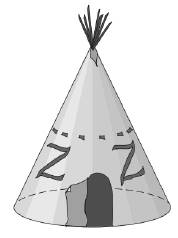Chapter 9.3, Problem 24E### Elementary Geometry for College St...

6th Edition
Daniel C. Alexander + 1 other
ISBN: 9781285195698

#### Solutions

Chapter
Section### Elementary Geometry for College St...

6th Edition
Daniel C. Alexander + 1 other
ISBN: 9781285195698
Textbook Problem
1 views

# Rukia discovers that the teepee with a circular floor has a radius length of 6 ft and a height of 15 ft. Find the volume of the enclosure.To determine

To find:

The volume of the enclosure.

Explanation

Given:

Rukia discovers that the teepee has a circular floor with a radius equal to 6 ft and a height of 15 ft.

Formula used:

The volume of the right circular cone is V=13πr2h.

Calculation:

Given, r=6ft and h=15ft.

Substitute the known values in the formula,

### Still sussing out bartleby?

Check out a sample textbook solution.

See a sample solution

#### The Solution to Your Study Problems

Bartleby provides explanations to thousands of textbook problems written by our experts, many with advanced degrees!

Get Started

#### Simplify: 5251155

Elementary Technical Mathematics

#### Evaluate the integrals in Problems 1-26.

Mathematical Applications for the Management, Life, and Social Sciences

#### Explain why plagiarism is unethical.

Research Methods for the Behavioral Sciences (MindTap Course List)

#### For j – 2k 3i – 4k 3i + j – 2k 0 (zero vector)

Study Guide for Stewart's Multivariable Calculus, 8th

#### The harmonic series is: 1 + 2 + 3 + 4 + …

Study Guide for Stewart's Single Variable Calculus: Early Transcendentals, 8th